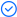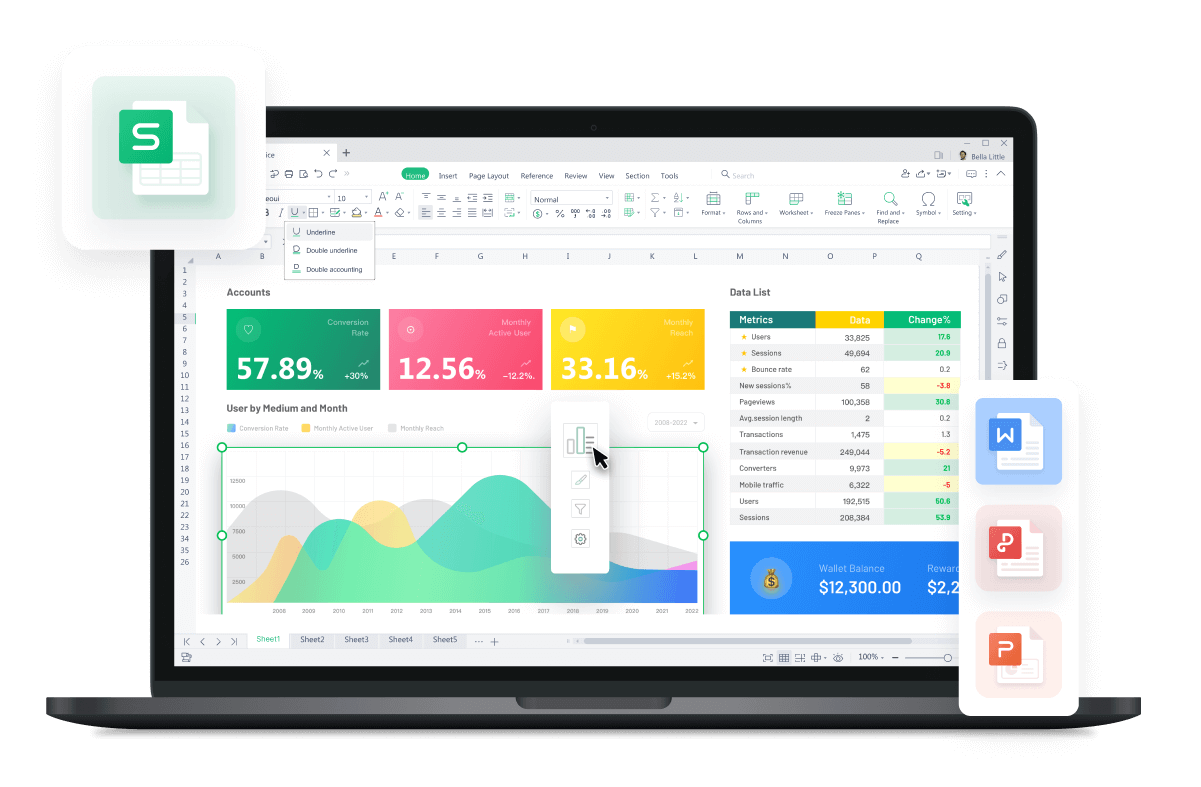WPS Office

Free All-in-One Office Suite with PDF EditorRead, edit, and convert PDFs with the powerful PDF toolkit.Microsoft-like interface, easy to use.

Windows • MacOS • Linux • iOS • Android# Easy Formula: How to use round function in excel

July 26, 2022
1.8K Views

Three functions to round numbers in Excel are demonstrated in this chapter. GO ROUND, GO ROUNDUP, and GO ROUNDDOWN. Before you begin: When you round a number, precision is lost. If you don't want this, change the number itself but show less decimal places.

How to use Round function in excel online, 2016 and 2019:

A number can be rounded in Excel to a certain number of digits using the ROUND function. The ROUND function makes up- or downward rounds. We round down 1, 2, 3, and 4. 5 through 9 are rounded up.

Round a number, for instance, to three decimal points.

1.It should be noted that 114.7261, 114.7262, 114.7263, and 114.7264 are rounded up to 114.727, while 114.7265, 114.7266, 114.7267, 114.7268, and 114.7269 are rounded down to 114.726.Two decimal places are used to round a number.

2.To the nearest decimal, round a number.

3.To the nearest whole number, round a number.

4.To the nearest ten, round a number.

5.Calculate a number to the closest hundred.

6.Calculate a number to the nearest thousand.

7.Negative numbers are rounded to one decimal place.

8.Negative numbers are rounded to the next whole number.

RoundUp in excel:Excel's ROUNDUP function always rounds up numbers (away from zero). Round up to the nearest 1, 2, 3, 4, 5, 6, 7, 8, and 9.Round a number, for instance, up to three decimal points.

1.It should be noted that 114.727 is rounded up to include 114.7261, 114.7262, 114.7263, 114.7264, 114.7265, 114.7266, 114.7267, 114.7268, and 114.7269.Two decimal places should be added to a number.

2.Raise a number's decimal point by one.

3.A number is rounded up to the next integer.

4.To the nearest ten, round a number up.

5.A number is rounded up to the nearest hundred.

6.A negative number should be rounded up one decimal place.

7.Keep in mind that the ROUNDUP function rounds up numbers (away from zero).Negative numbers are rounded up to the next integer.

Note that the ROUNDUP function once more rounds numbers upwards (away from zero).RoundDown in excel:In Excel, the ROUNDDOWN function, a number is always rounded down (toward zero). All numbers between 1 and 9 are rounded down.Round a number, for instance, to the third decimal place.

1.Two decimal places should be added to a number.

2.Round a number to the nearest tenth.

3.To the closest thousand, round a number down.

4.Negative numbers are rounded to the nearest decimal point.

5.A negative number should be rounded to the next integer.

Note: This above written article is an attempt to show you how to use the round function in excel online, 2016 and 2019, in both windows and mac.You just need to have a little understanding of how and which way things work and you are good to go. With having this basic knowledge or information of how to use it, you can also access and use different other options on excel or spreadsheet. Also, it is very similar to Word or Document. So, in a way, if you learn one thing, like Excel, you can automatically learn how to use Word as well because both of them are very similar in so many ways. If you want to know more about WPS Office, you can download WPS Office to access, Word, Excel, PowerPoint for free.